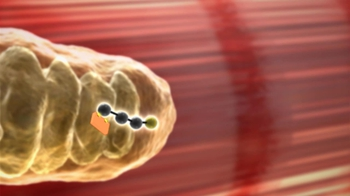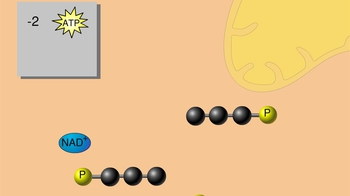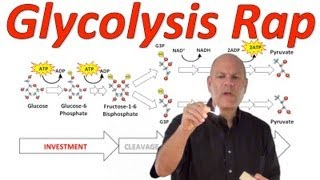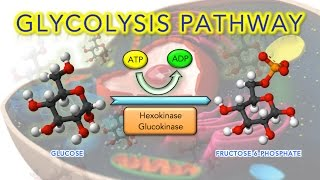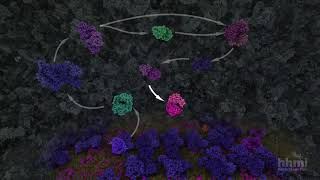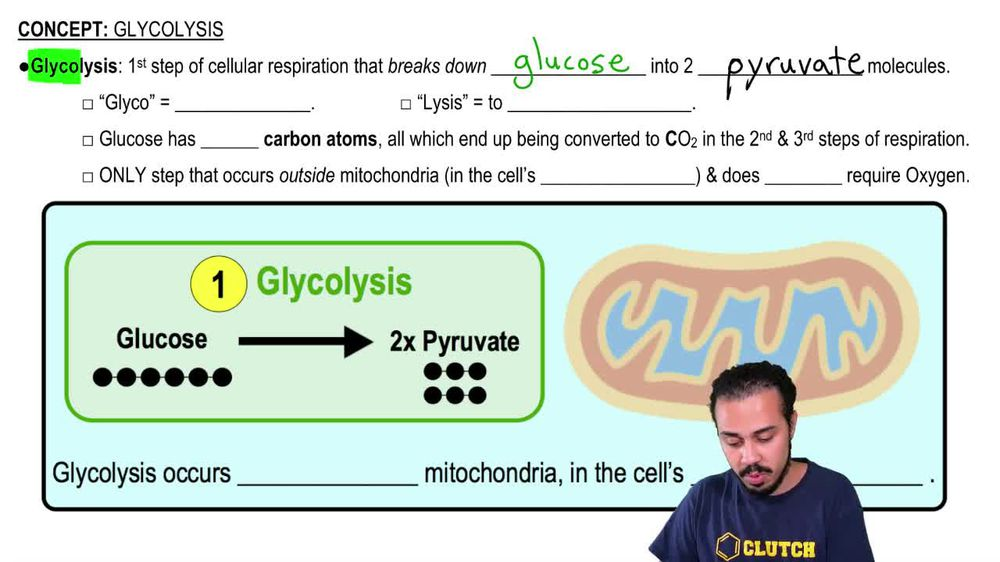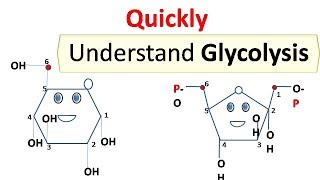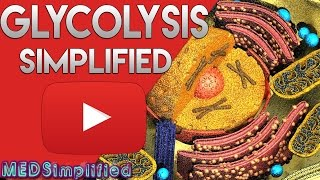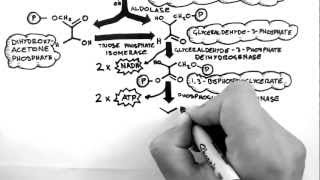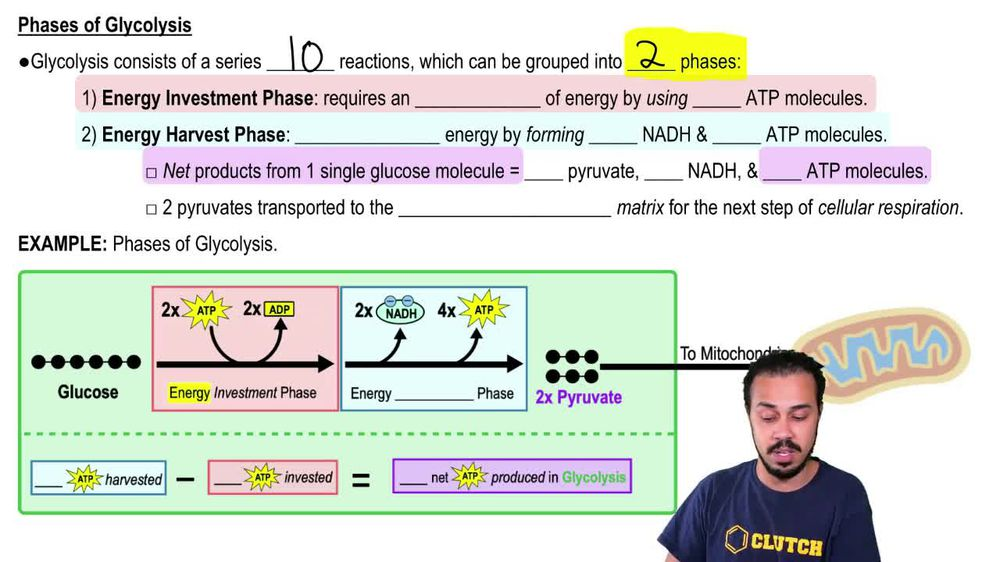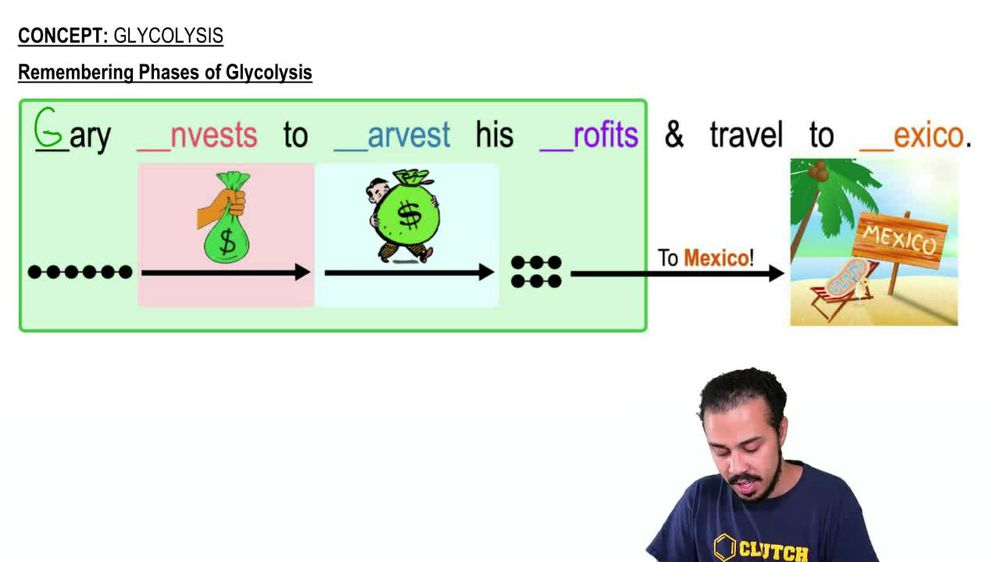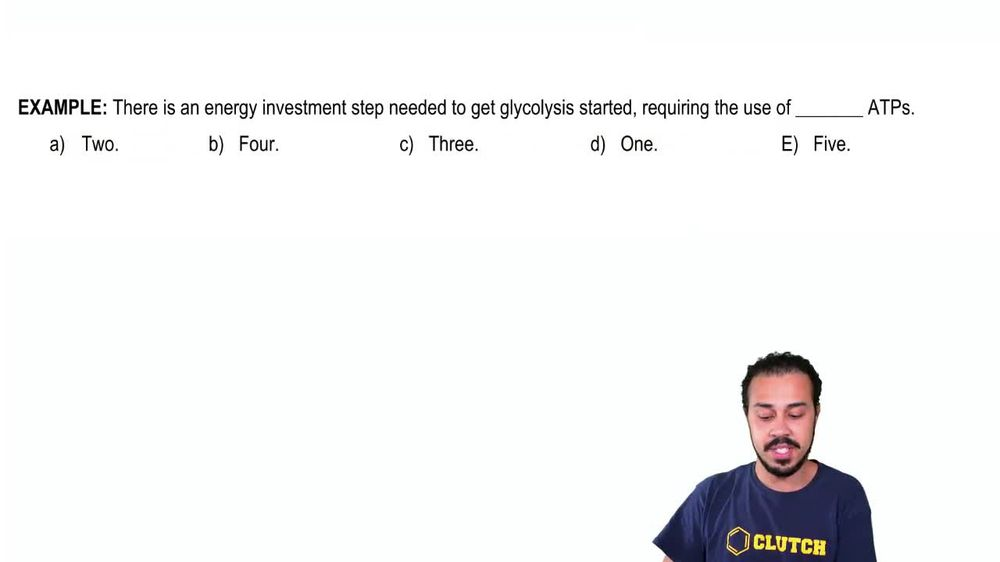Start typing, then use the up and down arrows to select an option from the list.
1. 8. Respiration2. Glycolysis# Glycolysis Example 1

by Jason Amores Sumpter
260 views
3
3
So here we have an example problem that wants us to fill in the blank here, using one of these five potential answer options down below And the example problem says there is an energy investment step needed to get Glen Collis has started requiring the use of Blank a TPS. And once again, we've got these five potential answer options down below. And so what we need to recall is that Glen Collis is has two phases the energy investment phase and the energy harvest phase. The energy investment phase requires an investment of 2. 80 p s. And so really, that's what this example problem is getting at an order for the energy investment step to occur, it requires the use of 2. 80 p s. And so the correct answer here is going to be answer option A. Now, after the 2 80 p s have been used, then the cell can transition to the second phase of Glen Collis is which is the energy harvest phase. And the energy harvest phase produces a total of 4 80 p s and two and a. D h. And of those 4. 80 p s because two of them got burnt. Ultimately, it's on Lee, a net of two ATP's that are made. But once again, in terms of the energy that needs to be invested, it's to a TPS that need to be invested. So the correct answer to this example is answer. Option A. And that concludes this example. So I'll see you all in our next video.